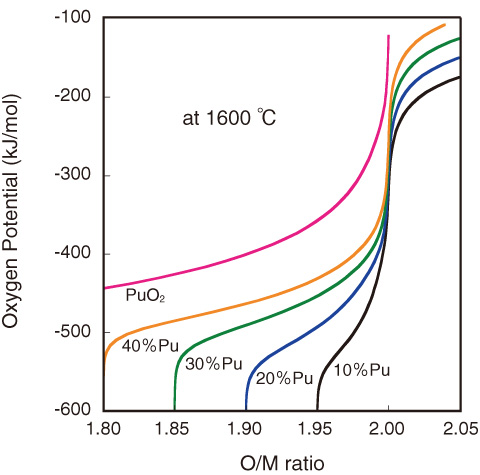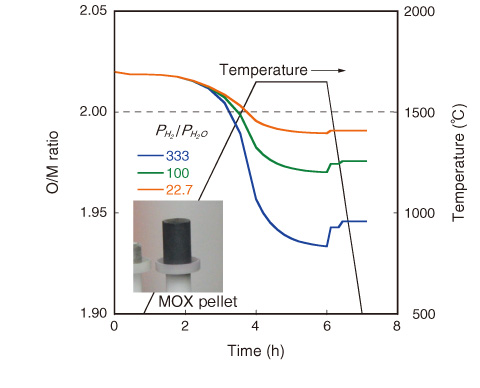# 2-6 Controlling Oxygen Content of U-Pu Mixed Oxide Fuel

## －Oxygen Potential Measurement and Application to Fuel Technologies－Fig.2-13　Oxygen potential of MOX at 1600 °C as functions of O/M ratio and Pu content

The O/M ratio decreases with decreasing oxygen potential and increasing Pu content. The O/M ratio can be controlled by controlling the oxygen potential and temperature.Fig.2-14　O/M change of (U0.7Pu0.3)O2-x during heat treatment

The O/M ratio of MOX changes with PH2 /PH2O ratio. The O/M ratio reaches 1.94 at 1650 °C in atmosphere with PH2 /PH2O = 333. Photograph shows MOX pellet set in the measurement system.

Uranium-plutonium mixed oxide (MOX) has been used as a fuel for sodium-cooled fast reactors; it has a fluorite structure with an oxygen-to-metal (O/M) ratio of 2.00. UO2 can take extra oxygen and exist in a hyper-stoichiometric composition with a range of O/M > 2.00. Pu atoms are substituted for U atoms, and a MOX solid solution is formed. On the other hand, MOX can exist in a hypo-stoichiometric region of O/M < 2.00 by forming oxygen vacancies. Changes in the O/M ratio significantly affect various properties of MOX, such as the thermal conductivity. Therefore, it is important to control the O/M ratio to develop fuel technologies.

It is necessary to know the relationship among the O/M ratio, temperature, and oxygen potential to develop O/M control techniques. In this work, the oxygen potential (ΔGO2) of MOX was measured by the gas equilibrium method. The oxygen potential is a quantity used in thermodynamics to describe the oxygen chemical stability in MOX and can be obtained using eq.(1).

ΔGO2 = RT lnPO2, .....(1)
where R is the gas constant. A gas mixture of Ar/H2 to add moisture was used for oxygen potential control. In this gas system, the oxygen potential is determined by the reaction H2O⇔H2+1/2O2 and controlled by adjusting the PH2/PH2O ratio. Many data (more than 1000 points) were obtained and evaluated, and an equation representing the oxygen potential was derived as a function of the O/M ratio, temperature, and Pu content. On the basis of this equation, a technique for evaluating the O/M ratio was developed.

Fig.2-13 shows the relationship between the oxygen potential and the O/M ratio at 1600 °C. The oxygen potential increases with increasing Pu content. This technology for evaluating the oxygen potential has been applied to the fuel pellet fabrication process and irradiation behavior analysis. The O/M change in (U0.7Pu0.3)O2-x during heat treatment is plotted as a function of the PH2 /PH2O ratio in Fig.2-14. The figure shows that the PH2 /PH2O ratio affects the variation in O/M. Further, the chemical stability of fission products inside irradiated pellets can be evaluated using this technology.

| | | | |